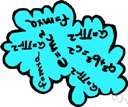# abelian group

(redirected from Abelian groups)
Also found in: Thesaurus, Encyclopedia.

## a·be·li·an group

(ə-bē′lē-ən)
n.

[After Niels Henrik Abel (1802-1829), Norwegian mathematician.]

## Abelian group

(əˈbiːlɪən)
n
(Mathematics) a group the defined binary operation of which is commutative: if a and b are members of an Abelian group then ab = ba
[C19: named after Niels Henrik Abel (1802–29), Norwegian mathematician]
Collins English Dictionary – Complete and Unabridged, 12th Edition 2014 © HarperCollins Publishers 1991, 1994, 1998, 2000, 2003, 2006, 2007, 2009, 2011, 2014
ThesaurusAntonymsRelated WordsSynonymsLegend:
 Noun 1Abelian group - a group that satisfies the commutative lawcommutative groupmathematical group, group - a set that is closed, associative, has an identity element and every element has an inverse
Based on WordNet 3.0, Farlex clipart collection. © 2003-2012 Princeton University, Farlex Inc.
Translations
Mentioned in ?
References in periodicals archive ?
Pollack introduces algebraic number theory to readers who are familiar with linear algebra, commutative ring theory, Galois theory, a little abelian groups theory, and elementary number theory up to and including the law of quadratic reciprocity.
Examples of such groups are: finite Abelian groups (, Theorem 4.2), generalized quaternion groups (, Corollary 1), torsion-free divisible Abelian groups (, Theorem 1), etc.
In fact for abelian groups (and much more general situations) we have by Schauenburg [Schau02] a Kunneth-type formula, and this decomposition does precisely explain the initially observed decomposition.
Let [H.sub.1], [H.sub.2] be two finite abelian groups and [f.sub.i] [member of] End([H.sub.i]).
Singh, "Some decomposition theorems in abelian groups and their generalizations," in Ring Theory: Proceedings of the Ohio University Conference, vol.
They discuss modules, including vector spaces and abelian groups, group theory, and quasigroups.
Lehmer, A ternary analogue of abelian groups, American Journal of Mathematics, 59 (1932) 329-338.
Among the topics are greatest common divisors, integer multiples and exponents, quotients of polynomial rings, divisibility and factorization in integral domains, subgroups of cyclic groups, cosets and Lagrange's theorem, the fundamental theorem of finite abelian groups, and check digits.
Theorem 1.1 (Fundamental theorem of finite abelian groups) Any finite abelian group G can be written as a direct sum of cyclic groups in the following canonical way: G = [MATHEMATICAL EXPRESSION NOT REPRODUCIBLE IN ASCII], where every [k.sub.i] (1 [less than or equal to] i [less than or equal to] l) is a prime power.
A quasi-arithmetic matroid endows a matroid with a multiplicity function, whose values (in the representable case) are the cardinalities of certain finite abelian groups, namely, the torsion parts of the quotients of an ambient lattice [Z.sup.n] by the sublattices spanned by subsets of vectors.

Site: Follow: Share:
Open / Close